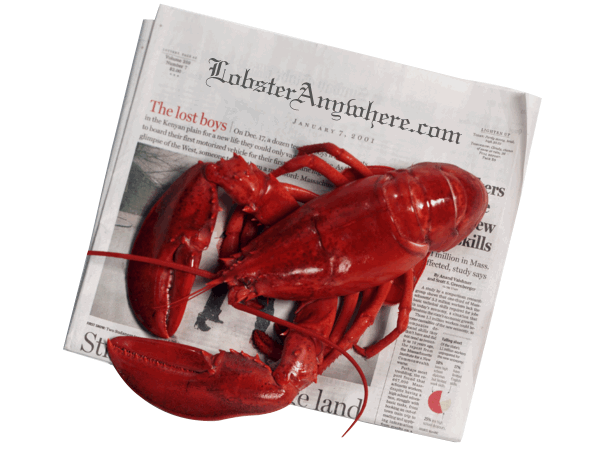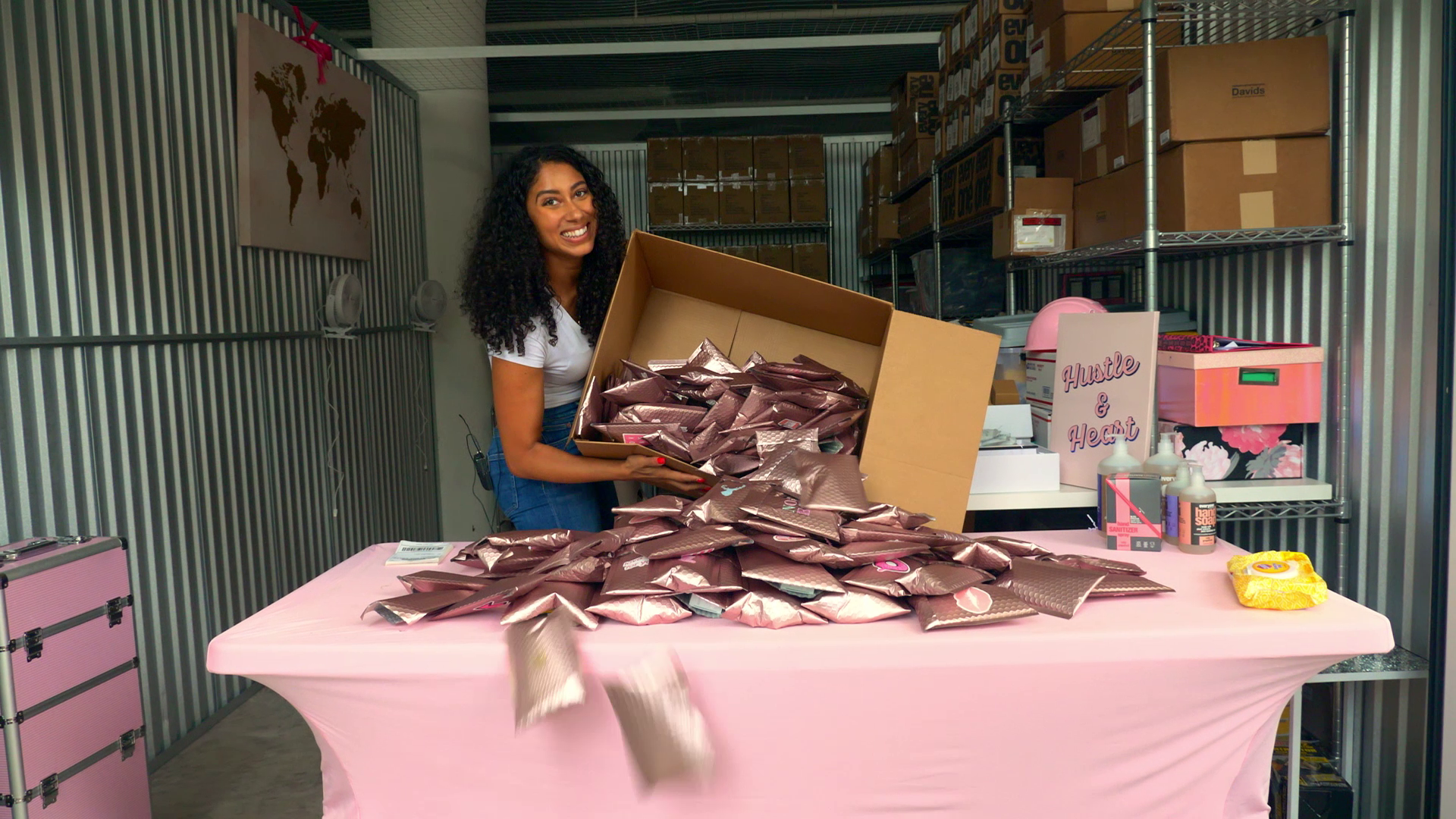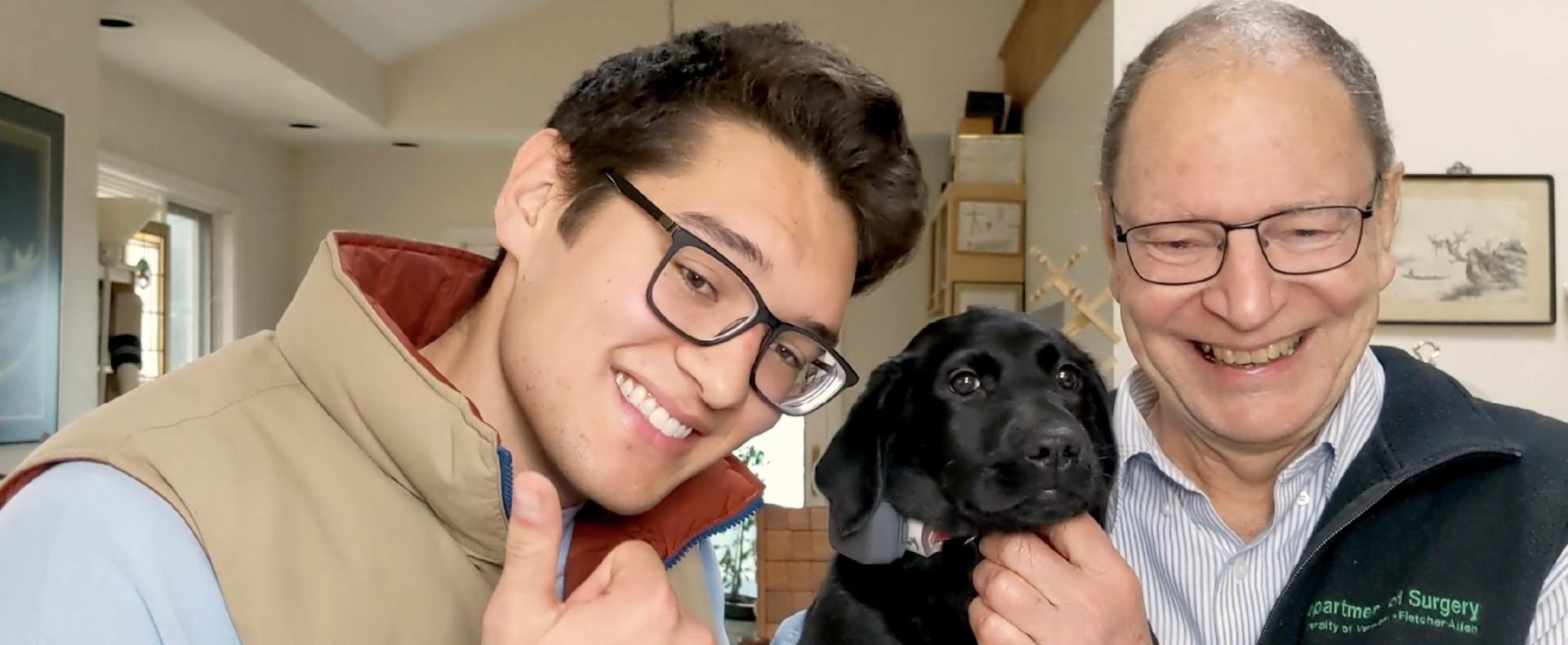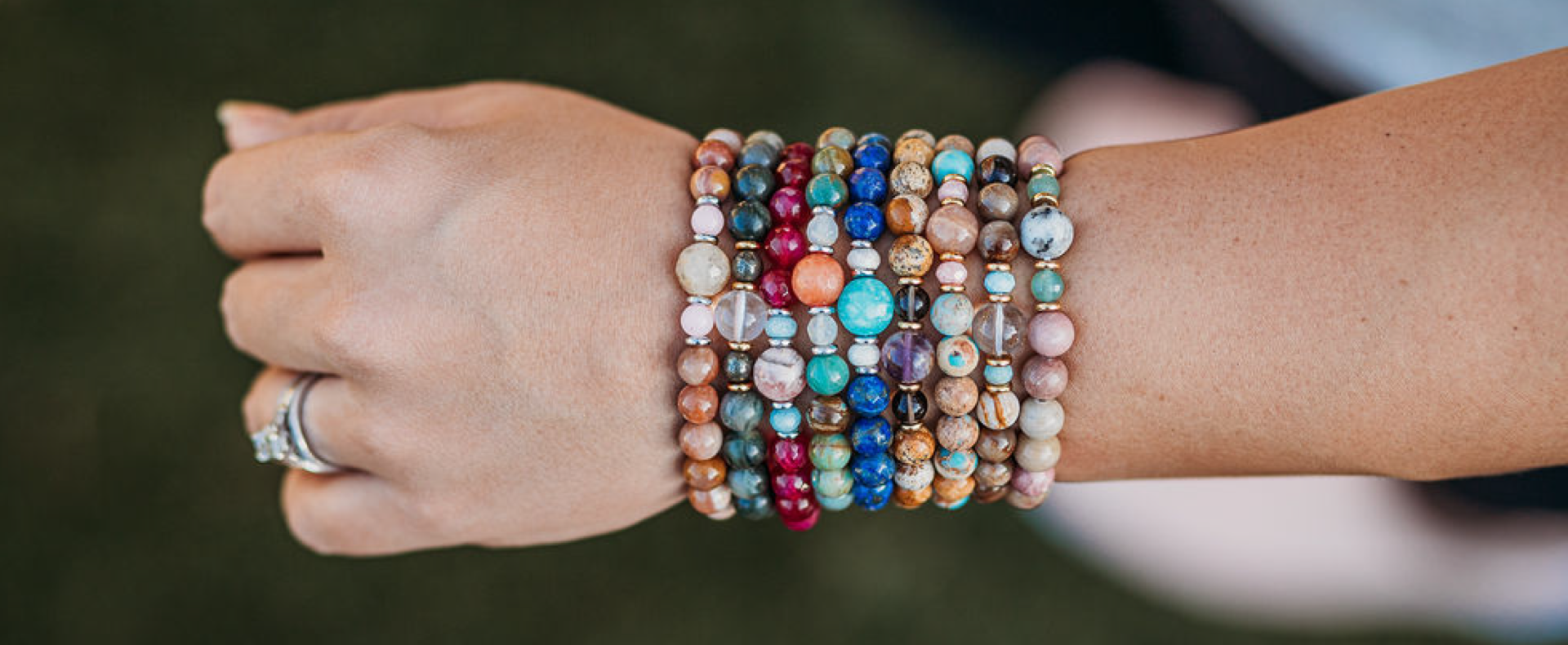# LobsterAnywhere

Published on November 21, 2015
Written by

### SUMMARY

Delivering live lobsters to your door has been the mission of LobsterAnywhere for years upon years. Learn how they keep their product fresh for your next feast.

### WHERE THEY SELL

www.LobsterAnywhere.com### I don’t think most people would expect Lobster Anywhere to be an ecommerce business, but you’re here, selling everything from live lobsters to filet mignon with fast delivery options. What was Lobster Anywhere’s journey to this point like?

We’ve been doing it too long, since 1999. We survived the dot com bust and market crashes and more. We have gained a pretty loyal customer base who have stuck with us and recommend us to family and friends.

Yes, lobster lovers and land lubbers all across the country are amazed we can ship <strong>live</strong> lobsters to their front doors, alive and fighting. You get what you pay for, they say. We start with great hard shell lobsters—there is a difference in quality. What we discovered through the years is that we just don’t ship over-the-top food, we ship a complete experience. We find only the best food to complement our lobster, like our chowder, steaks, crab—even a lobster roll kit. We offer a menu of seafood that many companies have tried to copy. Again, it comes down to quality in everything—and also local resources.

We are dedicated to lobster resource conservation and committed to maintaining a healthy sustainable resource.

### You include a “How-to-Cook-Lobster” guide with every live lobster—what spurred that decision?

A cooking and handling guide we offered right from the start but have made many improvements throughout the years. For example, some folks do not realize lobsters are a dark brown greenish color and only turn red when you cook them! We include tips for storing lobsters, how to cook lobster tails on the grill, and some nice original recipes. Again, it’s all about the experience!

``` How difficult has it been shipping fresh food? No matter how you look at it, it's a lot of work. Our packages are custom packaged to order. What's more, our packages and gifts are perishable and time-sensitive. Not only do we source and grade top quality lively lobsters, but we use superior packaging. Our coolers are for 2-3 days, however, we still ship overnight and express two day. We have shipped to HI many times and as far away as the North Pole, AK. No kidding! Plus our products look nice when you get them. A lady from Malden, MA called us last week asking how the lobsters would be shipped to CA. I said they will be great. A big seafood company in Boston she called said they were having issues of dead lobsters on delivery. I explained they were not shipping grade-A hard shell lobsters. How has ShipStation helped you with your business? Thanks to Ship Station, we can process orders faster than you can say Penobscot Bay. We do not ship widgets, we ship super fresh seafood. So getting it shipped and delivered on the right day and on time is a big deal. We are able to handle two day, overnight, early morning, and even Saturday shipping labels in a breeze. Plus, with your new mobile app, we can check the status of packages anywhere, even on the boat! And customers receive timely emails to let them know when to start boiling the water and get cracking. What's your favorite ShipStation feature? What's one the best and most overlooked features that many shipping programs lack is the ability to select a delivery date! If Uncle Phil's 50th birthday is on Tuesday we can schedule a nice 3 pound lobster and chowder to arrive ON Phil's big day.Joe Bowab President & Chief Lobster Shipper Plus, with your new mobile app, we can check the status of packages anywhere, even on the boat! And customers receive timely emails to let them know when to start boiling the water and get cracking.```
```Written by Joey I'm Joey, ShipStation's Content & Social Media Coordinator. I spent over a year on ShipStation's Support team which has given me great insight into our product and our customers. Outside of work, you'll find me writing, getting into one of Austin's great microbrews, or relaxing on a trail with my mutt. ```
``` SUMMARY Delivering live lobsters to your door has been the mission of LobsterAnywhere for years upon years. Learn how they keep their product fresh for your next feast. WHERE THEY SELL www.LobsterAnywhere.com ```
``` More in User Stories Browse AllThe Stay Beautiful Foundation "With ShipStation, I’m able to create 500 labels in less than a few minutes and get beauty in the hands of women who are battling cancer so much quicker. It allows me to put so much more time into the things that are really valuable to the Stay Beautiful Foundation and helps us grow and scale."Synergy "ShipStation integrates seamlessly into all new and emerging marketplaces. It allows us to focus less on shipping, and more on generating revenue for our clients."QOR360 "ShipStation and UPS allows us to scale and fulfill with the same quality as billion-dollar companies."The Groomsman Suit "ShipStation has one simple dashboard with all of our shipping needs. We can compare rates across different carriers, understand delivery time frames, and quote customers expedited shipping."Tout Cuit "I appreciate how intuitive and practical ShipStation's features are when processing delivery labels. It's really fast and efficient!"HorseFeathers Gifts "I’ve learned so much with ShipStation, it is seriously amazing. I tell everybody about how it will change your business and how efficiently you can run everything." ```
``` ```
``` ```
``` Want to learn more about ShipStation? View Features Schedule a Demo ```
```4301 Bull Creek Rd Austin, TX 78731 Copyright © 2011-2023 ShipStation Privacy Policy Do Not Sell My Personal Information Limit the Use of My Sensitive Personal Information Features Partners Pricing Heard Our Ad? Login Support About Us Careers Blog Resource Hub Newsroom Stories Videos Webinars Integrations Developers Affiliates Experts Contact Us;window.NREUM||(NREUM={});NREUM.init={distributed_tracing:{enabled:true},privacy:{cookies_enabled:true},ajax:{deny_list:["bam.nr-data.net"]}}; ;NREUM.loader_config={accountID:"3797132",trustKey:"1551542",agentID:"550266175",licenseKey:"NRJS-546049dfef97d987c57",applicationID:"550266175"} ;NREUM.info={beacon:"bam.nr-data.net",errorBeacon:"bam.nr-data.net",licenseKey:"NRJS-546049dfef97d987c57",applicationID:"550266175",sa:1} ;(()=>{var e,t,r={9071:(e,t,r)=>{"use strict";r.d(t,{I:()=>n});var n=0,i=navigator.userAgent.match(/Firefox[\/\s](\d+\.\d+)/);i&&(n=+i)},8768:(e,t,r)=>{"use strict";r.d(t,{T:()=>n,p:()=>i});const n=/(iPad|iPhone|iPod)/g.test(navigator.userAgent),i=n&&Boolean("undefined"==typeof SharedWorker)},2919:(e,t,r)=>{"use strict";r.d(t,{P_:()=>g,Mt:()=>v,C5:()=>c,DL:()=>y,OP:()=>R,lF:()=>P,Yu:()=>E,Dg:()=>p,CX:()=>u,GE:()=>w,sU:()=>C});var n={};r.r(n),r.d(n,{agent:()=>A,match:()=>N,version:()=>x});var i=r(6797),o=r(4286);const a={beacon:i.ce.beacon,errorBeacon:i.ce.errorBeacon,licenseKey:void 0,applicationID:void 0,sa:void 0,queueTime:void 0,applicationTime:void 0,ttGuid:void 0,user:void 0,account:void 0,product:void 0,extra:void 0,jsAttributes:{},userAttributes:void 0,atts:void 0,transactionName:void 0,tNamePlain:void 0},s={};function c(e){if(!e)throw new Error("All info objects require an agent identifier!");if(!s[e])throw new Error("Info for ".concat(e," was never set"));return s[e]}function u(e,t){if(!e)throw new Error("All info objects require an agent identifier!");s[e]=new o.I(t,a),(0,i.Qy)(e,s[e],"info")}var d=r(2797),f=r(2374);const l={allow_bfcache:!0,privacy:{cookies_enabled:!0},ajax:{deny_list:void 0,enabled:!0,harvestTimeSeconds:10},distributed_tracing:{enabled:void 0,exclude_newrelic_header:void 0,cors_use_newrelic_header:void 0,cors_use_tracecontext_headers:void 0,allowed_origins:void 0},session:{domain:void 0,expiresMs:d.oD,inactiveMs:d.Hb},ssl:void 0,obfuscate:void 0,jserrors:{enabled:!0,harvestTimeSeconds:10},metrics:{enabled:!0},page_action:{enabled:!0,harvestTimeSeconds:30},page_view_event:{enabled:!0},page_view_timing:{enabled:!0,harvestTimeSeconds:30,long_task:!1},session_trace:{enabled:!0,harvestTimeSeconds:10},spa:{enabled:!0,harvestTimeSeconds:10}},h={};function g(e){if(!e)throw new Error("All configuration objects require an agent identifier!");if(!h[e])throw new Error("Configuration for ".concat(e," was never set"));return h[e]}function p(e,t){if(!e)throw new Error("All configuration objects require an agent identifier!");h[e]=new o.I(t,l),(0,i.Qy)(e,h[e],"config")}function v(e,t){if(!e)throw new Error("All configuration objects require an agent identifier!");var r=g(e);if(r){for(var n=t.split("."),i=0;i<n.length-1;i++)if("object"!=typeof(r=r[n[i]]))return;r=r[n[n.length-1]]}return r}const m={accountID:void 0,trustKey:void 0,agentID:void 0,licenseKey:void 0,applicationID:void 0,xpid:void 0},b={};function y(e){if(!e)throw new Error("All loader-config objects require an agent identifier!");if(!b[e])throw new Error("LoaderConfig for ".concat(e," was never set"));return b[e]}function w(e,t){if(!e)throw new Error("All loader-config objects require an agent identifier!");b[e]=new o.I(t,m),(0,i.Qy)(e,b[e],"loader_config")}const E=(0,i.mF)().o;var A=null,x=null;const T=/Version\/(\S+)\s+Safari/;if(navigator.userAgent){var _=navigator.userAgent,D=_.match(T);D&&-1===_.indexOf("Chrome")&&-1===_.indexOf("Chromium")&&(A="Safari",x=D)}function N(e,t){if(!A)return!1;if(e!==A)return!1;if(!t)return!0;if(!x)return!1;for(var r=x.split("."),n=t.split("."),i=0;i<n.length;i++)if(n[i]!==r[i])return!1;return!0}var S=r(1651);const O={buildEnv:S.Re,bytesSent:{},queryBytesSent:{},customTransaction:void 0,disabled:!1,distMethod:S.gF,isolatedBacklog:!1,loaderType:void 0,maxBytes:3e4,offset:Math.floor(f._A?.performance?.timeOrigin||f._A?.performance?.timing?.navigationStart||Date.now()),onerror:void 0,origin:""+f._A.location,ptid:void 0,releaseIds:{},session:void 0,xhrWrappable:"function"==typeof f._A.XMLHttpRequest?.prototype?.addEventListener,userAgent:n,version:S.q4},j={};function R(e){if(!e)throw new Error("All runtime objects require an agent identifier!");if(!j[e])throw new Error("Runtime for ".concat(e," was never set"));return j[e]}function C(e,t){if(!e)throw new Error("All runtime objects require an agent identifier!");j[e]=new o.I(t,O),(0,i.Qy)(e,j[e],"runtime")}function P(e){return function(e){try{const t=c(e);return!!t.licenseKey&&!!t.errorBeacon&&!!t.applicationID}catch(e){return!1}}(e)}},4286:(e,t,r)=>{"use strict";r.d(t,{I:()=>o});var n=r(909),i=r(8610);class o{constructor(e,t){Object.assign(this,function(e,t){const r={};try{return e&&"object"==typeof e?t&&"object"==typeof t?(Object.assign(r,t),Object.entries(e).forEach((e=>{let[o,a]=e;if(!Object.keys(t).includes(o))return;const s=(0,n.q)(o);s.length&&a&&"object"==typeof a&&s.forEach((e=>{e in a&&((0,i.Z)('"'.concat(e,'" is a protected attribute and can not be changed in feature ').concat(o,". It will have no effect.")),delete a[e])})),r[o]=a})),r):(0,i.Z)("Setting a Configurable requires a model to set its initial properties"):(0,i.Z)("New setting a Configurable requires an object as input")}catch(e){(0,i.Z)("An error occured while setting a Configurable",e)}}(e,t))}}},1651:(e,t,r)=>{"use strict";r.d(t,{Re:()=>i,gF:()=>o,q4:()=>n});const n="1.232.0",i="PROD",o="CDN"},9557:(e,t,r)=>{"use strict";r.d(t,{w:()=>o});var n=r(8610);const i={agentIdentifier:"",ee:void 0};class o{constructor(e){try{if("object"!=typeof e)return(0,n.Z)("shared context requires an object as input");this.sharedContext={},Object.assign(this.sharedContext,i),Object.entries(e).forEach((e=>{let[t,r]=e;Object.keys(i).includes(t)&&(this.sharedContext[t]=r)}))}catch(e){(0,n.Z)("An error occured while setting SharedContext",e)}}}},4329:(e,t,r)=>{"use strict";r.d(t,{L:()=>d,R:()=>c});var n=r(3752),i=r(7022),o=r(4045),a=r(2325);const s={};function c(e,t){const r={staged:!1,priority:a.p[t]||0};u(e),s[e].get(t)||s[e].set(t,r)}function u(e){e&&(s[e]||(s[e]=new Map))}function d(){let e=arguments.length>0&&void 0!==arguments?arguments:"",t=arguments.length>1&&void 0!==arguments?arguments:"feature";if(u(e),!e||!s[e].get(t))return a(t);s[e].get(t).staged=!0;const r=Array.from(s[e]);function a(t){const r=e?n.ee.get(e):n.ee,a=o.X.handlers;if(r.backlog&&a){var s=r.backlog[t],c=a[t];if(c){for(var u=0;s&&u<s.length;++u)f(s[u],c);(0,i.D)(c,(function(e,t){(0,i.D)(t,(function(t,r){r.on(e,r)}))}))}delete a[t],r.backlog[t]=null,r.emit("drain-"+t,[])}}r.every((e=>{let[t,r]=e;return r.staged}))&&(r.sort(((e,t)=>e.priority-t.priority)),r.forEach((e=>{let[t]=e;a(t)})))}function f(e,t){var r=e;(0,i.D)(t[r],(function(t,r){var n=e;if(r===n){var i=r,o=e,a=e;i.apply(o,a)}}))}},3752:(e,t,r)=>{"use strict";r.d(t,{c:()=>f,ee:()=>u});var n=r(6797),i=r(3916),o=r(7022),a=r(2919),s="nr@context";let c=(0,n.fP)();var u;function d(){}function f(e){return(0,i.X)(e,s,l)}function l(){return new d}function h(){u.aborted=!0,u.backlog={}}c.ee?u=c.ee:(u=function e(t,r){var n={},c={},f={},g=!1;try{g=16===r.length&&(0,a.OP)(r).isolatedBacklog}catch(e){}var p={on:b,addEventListener:b,removeEventListener:y,emit:m,get:E,listeners:w,context:v,buffer:A,abort:h,aborted:!1,isBuffering:x,debugId:r,backlog:g?{}:t&&"object"==typeof t.backlog?t.backlog:{}};return p;function v(e){return e&&e instanceof d?e:e?(0,i.X)(e,s,l):l()}function m(e,r,n,i,o){if(!1!==o&&(o=!0),!u.aborted||i){t&&o&&t.emit(e,r,n);for(var a=v(n),s=w(e),d=s.length,f=0;f<d;f++)s[f].apply(a,r);var l=T()[c[e]];return l&&l.push([p,e,r,a]),a}}function b(e,t){n[e]=w(e).concat(t)}function y(e,t){var r=n[e];if(r)for(var i=0;i<r.length;i++)r[i]===t&&r.splice(i,1)}function w(e){return n[e]||[]}function E(t){return f[t]=f[t]||e(p,t)}function A(e,t){var r=T();p.aborted||(0,o.D)(e,(function(e,n){t=t||"feature",c[n]=t,t in r||(r[t]=[])}))}function x(e){return!!T()[c[e]]}function T(){return p.backlog}}(void 0,"globalEE"),c.ee=u)},9252:(e,t,r)=>{"use strict";r.d(t,{E:()=>n,p:()=>i});var n=r(3752).ee.get("handle");function i(e,t,r,i,o){o?(o.buffer([e],i),o.emit(e,t,r)):(n.buffer([e],i),n.emit(e,t,r))}},4045:(e,t,r)=>{"use strict";r.d(t,{X:()=>o});var n=r(9252);o.on=a;var i=o.handlers={};function o(e,t,r,o){a(o||n.E,i,e,t,r)}function a(e,t,r,i,o){o||(o="feature"),e||(e=n.E);var a=t[o]=t[o]||{};(a[r]=a[r]||[]).push([e,i])}},8544:(e,t,r)=>{"use strict";r.d(t,{bP:()=>s,iz:()=>c,m\$:()=>a});var n=r(2374);let i=!1,o=!1;try{const e={get passive(){return i=!0,!1},get signal(){return o=!0,!1}};n._A.addEventListener("test",null,e),n._A.removeEventListener("test",null,e)}catch(e){}function a(e,t){return i||o?{capture:!!e,passive:i,signal:t}:!!e}function s(e,t){let r=arguments.length>2&&void 0!==arguments&&arguments,n=arguments.length>3?arguments:void 0;window.addEventListener(e,t,a(r,n))}function c(e,t){let r=arguments.length>2&&void 0!==arguments&&arguments,n=arguments.length>3?arguments:void 0;document.addEventListener(e,t,a(r,n))}},5526:(e,t,r)=>{"use strict";r.d(t,{Ht:()=>u,M:()=>c,Rl:()=>a,ky:()=>s});var n=r(2374);const i="xxxxxxxx-xxxx-4xxx-yxxx-xxxxxxxxxxxx";function o(e,t){return e?15&e[t]:16*Math.random()|0}function a(){const e=n._A?.crypto||n._A?.msCrypto;let t,r=0;return e&&e.getRandomValues&&(t=e.getRandomValues(new Uint8Array(31))),i.split("").map((e=>"x"===e?o(t,++r).toString(16):"y"===e?(3&o()|8).toString(16):e)).join("")}function s(e){const t=n._A?.crypto||n._A?.msCrypto;let r,i=0;t&&t.getRandomValues&&(r=t.getRandomValues(new Uint8Array(31)));const a=[];for(var s=0;s<e;s++)a.push(o(r,++i).toString(16));return a.join("")}function c(){return s(16)}function u(){return s(32)}},2797:(e,t,r)=>{"use strict";r.d(t,{Bq:()=>n,Hb:()=>o,oD:()=>i});const n="NRBA",i=144e5,o=18e5},2053:(e,t,r)=>{"use strict";function n(){return Math.round(performance.now())}r.d(t,{z:()=>n})},6368:(e,t,r)=>{"use strict";r.d(t,{e:()=>o});var n=r(2374),i={};function o(e){if(e in i)return i[e];if(0===(e||"").indexOf("data:"))return{protocol:"data"};let t;var r=n._A?.location,o={};if(n.il)t=document.createElement("a"),t.href=e;else try{t=new URL(e,r.href)}catch(e){return o}o.port=t.port;var a=t.href.split("://");!o.port&&a&&(o.port=a.split("/").split("@").pop().split(":")),o.port&&"0"!==o.port||(o.port="https"===a?"443":"80"),o.hostname=t.hostname||r.hostname,o.pathname=t.pathname,o.protocol=a,"/"!==o.pathname.charAt(0)&&(o.pathname="/"+o.pathname);var s=!t.protocol||":"===t.protocol||t.protocol===r.protocol,c=t.hostname===r.hostname&&t.port===r.port;return o.sameOrigin=s&&(!t.hostname||c),"/"===o.pathname&&(i[e]=o),o}},8610:(e,t,r)=>{"use strict";function n(e,t){"function"==typeof console.warn&&(console.warn("New Relic: ".concat(e)),t&&console.warn(t))}r.d(t,{Z:()=>n})},3916:(e,t,r)=>{"use strict";r.d(t,{X:()=>i});var n=Object.prototype.hasOwnProperty;function i(e,t,r){if(n.call(e,t))return e[t];var i=r();if(Object.defineProperty&&Object.keys)try{return Object.defineProperty(e,t,{value:i,writable:!0,enumerable:!1}),i}catch(e){}return e[t]=i,i}},2374:(e,t,r)=>{"use strict";r.d(t,{_A:()=>o,il:()=>n,v6:()=>i});const n=Boolean("undefined"!=typeof window&&window.document),i=Boolean("undefined"!=typeof WorkerGlobalScope&&self.navigator instanceof WorkerNavigator);let o=(()=>{if(n)return window;if(i){if("undefined"!=typeof globalThis&&globalThis instanceof WorkerGlobalScope)return globalThis;if(self instanceof WorkerGlobalScope)return self}throw new Error('New Relic browser agent shutting down due to error: Unable to locate global scope. This is possibly due to code redefining browser global variables like "self" and "window".')})()},7022:(e,t,r)=>{"use strict";r.d(t,{D:()=>n});const n=(e,t)=>Object.entries(e||{}).map((e=>{let[r,n]=e;return t(r,n)}))},2438:(e,t,r)=>{"use strict";r.d(t,{P:()=>o});var n=r(3752);const i=()=>{const e=new WeakSet;return(t,r)=>{if("object"==typeof r&&null!==r){if(e.has(r))return;e.add(r)}return r}};function o(e){try{return JSON.stringify(e,i())}catch(e){try{n.ee.emit("internal-error",[e])}catch(e){}}}},2650:(e,t,r)=>{"use strict";r.d(t,{K:()=>a,b:()=>o});var n=r(8544);function i(){return"undefined"==typeof document||"complete"===document.readyState}function o(e,t){if(i())return e();(0,n.bP)("load",e,t)}function a(e){if(i())return e();(0,n.iz)("DOMContentLoaded",e)}},6797:(e,t,r)=>{"use strict";r.d(t,{EZ:()=>u,Qy:()=>c,ce:()=>o,fP:()=>a,gG:()=>d,mF:()=>s});var n=r(2053),i=r(2374);const o={beacon:"bam.nr-data.net",errorBeacon:"bam.nr-data.net"};function a(){return i._A.NREUM||(i._A.NREUM={}),void 0===i._A.newrelic&&(i._A.newrelic=i._A.NREUM),i._A.NREUM}function s(){let e=a();return e.o||(e.o={ST:i._A.setTimeout,SI:i._A.setImmediate,CT:i._A.clearTimeout,XHR:i._A.XMLHttpRequest,REQ:i._A.Request,EV:i._A.Event,PR:i._A.Promise,MO:i._A.MutationObserver,FETCH:i._A.fetch}),e}function c(e,t,r){let i=a();const o=i.initializedAgents||{},s=o[e]||{};return Object.keys(s).length||(s.initializedAt={ms:(0,n.z)(),date:new Date}),i.initializedAgents={...o,[e]:{...s,[r]:t}},i}function u(e,t){a()[e]=t}function d(){return function(){let e=a();const t=e.info||{};e.info={beacon:o.beacon,errorBeacon:o.errorBeacon,...t}}(),function(){let e=a();const t=e.init||{};e.init={...t}}(),s(),function(){let e=a();const t=e.loader_config||{};e.loader_config={...t}}(),a()}},6998:(e,t,r)=>{"use strict";r.d(t,{N:()=>i});var n=r(8544);function i(e){let t=arguments.length>1&&void 0!==arguments&&arguments,r=arguments.length>2?arguments:void 0,i=arguments.length>3?arguments:void 0;return void(0,n.iz)("visibilitychange",(function(){if(t)return void("hidden"==document.visibilityState&&e());e(document.visibilityState)}),r,i)}},6408:(e,t,r)=>{"use strict";r.d(t,{W:()=>i});var n=r(2374);function i(){return"function"==typeof n._A?.PerformanceObserver}},7264:(e,t,r)=>{"use strict";r.d(t,{em:()=>y,u5:()=>O,QU:()=>C,_L:()=>H,Gm:()=>B,Lg:()=>F,gy:()=>G,BV:()=>K,Kf:()=>re});var n=r(3752),i=r(8683),o=r.n(i);const a="nr@original";var s=Object.prototype.hasOwnProperty,c=!1;function u(e,t){return e||(e=n.ee),r.inPlace=function(e,t,n,i,o){n||(n="");var a,s,c,u="-"===n.charAt(0);for(c=0;c<t.length;c++)l(a=e[s=t[c]])||(e[s]=r(a,u?s+n:n,i,s,o))},r.flag=a,r;function r(t,r,n,s,c){return l(t)?t:(r||(r=""),nrWrapper[a]=t,f(t,nrWrapper,e),nrWrapper);function nrWrapper(){var a,u,f,l;try{u=this,a=o()(arguments),f="function"==typeof n?n(a,u):n||{}}catch(t){d([t,"",[a,u,s],f],e)}i(r+"start",[a,u,s],f,c);try{return l=t.apply(u,a)}catch(e){throw i(r+"err",[a,u,e],f,c),e}finally{i(r+"end",[a,u,l],f,c)}}}function i(r,n,i,o){if(!c||t){var a=c;c=!0;try{e.emit(r,n,i,t,o)}catch(t){d([t,r,n,i],e)}c=a}}}function d(e,t){t||(t=n.ee);try{t.emit("internal-error",e)}catch(e){}}function f(e,t,r){if(Object.defineProperty&&Object.keys)try{return Object.keys(e).forEach((function(r){Object.defineProperty(t,r,{get:function(){return e[r]},set:function(t){return e[r]=t,t}})})),t}catch(e){d([e],r)}for(var n in e)s.call(e,n)&&(t[n]=e[n]);return t}function l(e){return!(e&&e instanceof Function&&e.apply&&!e[a])}var h=r(3916),g=r(2374);const p={},v=XMLHttpRequest,m="addEventListener",b="removeEventListener";function y(e){var t=function(e){return(e||n.ee).get("events")}(e);if(p[t.debugId]++)return t;p[t.debugId]=1;var r=u(t,!0);function i(e){r.inPlace(e,[m,b],"-",o)}function o(e,t){return e}return"getPrototypeOf"in Object&&(g.il&&w(document,i),w(g._A,i),w(v.prototype,i)),t.on(m+"-start",(function(e,t){var n=e;if(null!==n&&("function"==typeof n||"object"==typeof n)){var i=(0,h.X)(n,"nr@wrapped",(function(){var e={object:function(){if("function"!=typeof n.handleEvent)return;return n.handleEvent.apply(n,arguments)},function:n}[typeof n];return e?r(e,"fn-",null,e.name||"anonymous"):n}));this.wrapped=e=i}})),t.on(b+"-start",(function(e){e=this.wrapped||e})),t}function w(e,t){let r=e;for(;"object"==typeof r&&!Object.prototype.hasOwnProperty.call(r,m);)r=Object.getPrototypeOf(r);for(var n=arguments.length,i=new Array(n>2?n-2:0),o=2;o<n;o++)i[o-2]=arguments[o];r&&t(r,...i)}var E="fetch-",A=E+"body-",x=["arrayBuffer","blob","json","text","formData"],T=g._A.Request,_=g._A.Response,D="prototype",N="nr@context";const S={};function O(e){const t=function(e){return(e||n.ee).get("fetch")}(e);if(!(T&&_&&g._A.fetch))return t;if(S[t.debugId]++)return t;function r(e,r,n){var i=e[r];"function"==typeof i&&(e[r]=function(){var e,r=o()(arguments),a={};t.emit(n+"before-start",[r],a),a[N]&&a[N].dt&&(e=a[N].dt);var s=i.apply(this,r);return t.emit(n+"start",[r,e],s),s.then((function(e){return t.emit(n+"end",[null,e],s),e}),(function(e){throw t.emit(n+"end",[e],s),e}))})}return S[t.debugId]=1,x.forEach((e=>{r(T[D],e,A),r(_[D],e,A)})),r(g._A,"fetch",E),t.on(E+"end",(function(e,r){var n=this;if(r){var i=r.headers.get("content-length");null!==i&&(n.rxSize=i),t.emit(E+"done",[null,r],n)}else t.emit(E+"done",[e],n)})),t}const j={},R=["pushState","replaceState"];function C(e){const t=function(e){return(e||n.ee).get("history")}(e);return!g.il||j[t.debugId]++||(j[t.debugId]=1,u(t).inPlace(window.history,R,"-")),t}var P=r(8544);const I={},k=["appendChild","insertBefore","replaceChild"];function H(e){const t=function(e){return(e||n.ee).get("jsonp")}(e);if(!g.il||I[t.debugId])return t;I[t.debugId]=!0;var r=u(t),i=/[?&](?:callback|cb)=([^&#]+)/,o=/(.*)\.([^.]+)/,a=/^(\w+)(\.|\$)(.*)\$/;function s(e,t){var r=e.match(a),n=r,i=r;return i?s(i,t[n]):t[n]}return r.inPlace(Node.prototype,k,"dom-"),t.on("dom-start",(function(e){!function(e){if(!e||"string"!=typeof e.nodeName||"script"!==e.nodeName.toLowerCase())return;if("function"!=typeof e.addEventListener)return;var n=(a=e.src,c=a.match(i),c?c:null);var a,c;if(!n)return;var u=function(e){var t=e.match(o);if(t&&t.length>=3)return{key:t,parent:s(t,window)};return{key:e,parent:window}}(n);if("function"!=typeof u.parent[u.key])return;var d={};function f(){t.emit("jsonp-end",[],d),e.removeEventListener("load",f,(0,P.m\$)(!1)),e.removeEventListener("error",l,(0,P.m\$)(!1))}function l(){t.emit("jsonp-error",[],d),t.emit("jsonp-end",[],d),e.removeEventListener("load",f,(0,P.m\$)(!1)),e.removeEventListener("error",l,(0,P.m\$)(!1))}r.inPlace(u.parent,[u.key],"cb-",d),e.addEventListener("load",f,(0,P.m\$)(!1)),e.addEventListener("error",l,(0,P.m\$)(!1)),t.emit("new-jsonp",[e.src],d)}(e)})),t}var L=r(2919);const z={};function B(e){const t=function(e){return(e||n.ee).get("mutation")}(e);if(!g.il||z[t.debugId])return t;z[t.debugId]=!0;var r=u(t),i=L.Yu.MO;return i&&(window.MutationObserver=function(e){return this instanceof i?new i(r(e,"fn-")):i.apply(this,arguments)},MutationObserver.prototype=i.prototype),t}const M={};function F(e){const t=function(e){return(e||n.ee).get("promise")}(e);if(M[t.debugId])return t;M[t.debugId]=!0;var r=n.c,i=u(t),o=L.Yu.PR;return o&&function(){function e(r){var n=t.context(),a=i(r,"executor-",n,null,!1);const s=Reflect.construct(o,[a],e);return t.context(s).getCtx=function(){return n},s}g._A.Promise=e,Object.defineProperty(e,"name",{value:"Promise"}),e.toString=function(){return o.toString()},Object.setPrototypeOf(e,o),["all","race"].forEach((function(r){const n=o[r];e[r]=function(e){let i=!1;Array.from(e||[]).forEach((e=>{this.resolve(e).then(a("all"===r),a(!1))}));const o=n.apply(this,arguments);return o;function a(e){return function(){t.emit("propagate",[null,!i],o,!1,!1),i=i||!e}}}})),["resolve","reject"].forEach((function(r){const n=o[r];e[r]=function(e){const r=n.apply(this,arguments);return e!==r&&t.emit("propagate",[e,!0],r,!1,!1),r}})),e.prototype=o.prototype;const n=o.prototype.then;o.prototype.then=function(){var e=this,o=r(e);o.promise=e;for(var a=arguments.length,s=new Array(a),c=0;c<a;c++)s[c]=arguments[c];s=i(s,"cb-",o,null,!1),s=i(s,"cb-",o,null,!1);const u=n.apply(this,s);return o.nextPromise=u,t.emit("propagate",[e,!0],u,!1,!1),u},o.prototype.then[a]=n,t.on("executor-start",(function(e){e=i(e,"resolve-",this,null,!1),e=i(e,"resolve-",this,null,!1)})),t.on("executor-err",(function(e,t,r){e(r)})),t.on("cb-end",(function(e,r,n){t.emit("propagate",[n,!0],this.nextPromise,!1,!1)})),t.on("propagate",(function(e,r,n){this.getCtx&&!r||(this.getCtx=function(){if(e instanceof Promise)var r=t.context(e);return r&&r.getCtx?r.getCtx():this})}))}(),t}const U={},q="requestAnimationFrame";function G(e){const t=function(e){return(e||n.ee).get("raf")}(e);if(!g.il||U[t.debugId]++)return t;U[t.debugId]=1;var r=u(t);return r.inPlace(window,[q],"raf-"),t.on("raf-start",(function(e){e=r(e,"fn-")})),t}const V={},W="setTimeout",X="setInterval",Z="clearTimeout",\$="-start",Y="-",Q=[W,"setImmediate",X,Z,"clearImmediate"];function K(e){const t=function(e){return(e||n.ee).get("timer")}(e);if(V[t.debugId]++)return t;V[t.debugId]=1;var r=u(t);return r.inPlace(g._A,Q.slice(0,2),W+Y),r.inPlace(g._A,Q.slice(2,3),X+Y),r.inPlace(g._A,Q.slice(3),Z+Y),t.on(X+\$,(function(e,t,n){e=r(e,"fn-",null,n)})),t.on(W+\$,(function(e,t,n){this.method=n,this.timerDuration=isNaN(e)?0:+e,e=r(e,"fn-",this,n)})),t}var J=r(8610);const ee={},te=["open","send"];function re(e){var t=e||n.ee;const r=function(e){return(e||n.ee).get("xhr")}(t);if(ee[r.debugId]++)return r;ee[r.debugId]=1,y(t);var i=u(r),o=L.Yu.XHR,a=L.Yu.MO,s=L.Yu.PR,c=L.Yu.SI,d="readystatechange",f=["onload","onerror","onabort","onloadstart","onloadend","onprogress","ontimeout"],l=[],h=g._A.XMLHttpRequest.listeners,p=g._A.XMLHttpRequest=function(e){var t=new o(e);function n(){try{r.emit("new-xhr",[t],t),t.addEventListener(d,m,(0,P.m\$)(!1))}catch(e){(0,J.Z)("An error occured while intercepting XHR",e);try{r.emit("internal-error",[e])}catch(e){}}}return this.listeners=h?[...h,n]:[n],this.listeners.forEach((e=>e())),t};function v(e,t){i.inPlace(t,["onreadystatechange"],"fn-",x)}function m(){var e=this,t=r.context(e);e.readyState>3&&!t.resolved&&(t.resolved=!0,r.emit("xhr-resolved",[],e)),i.inPlace(e,f,"fn-",x)}if(function(e,t){for(var r in e)t[r]=e[r]}(o,p),p.prototype=o.prototype,i.inPlace(p.prototype,te,"-xhr-",x),r.on("send-xhr-start",(function(e,t){v(e,t),function(e){l.push(e),a&&(b?b.then(A):c?c(A):(w=-w,E.data=w))}(t)})),r.on("open-xhr-start",v),a){var b=s&&s.resolve();if(!c&&!s){var w=1,E=document.createTextNode(w);new a(A).observe(E,{characterData:!0})}}else t.on("fn-end",(function(e){e&&e.type===d||A()}));function A(){for(var e=0;e<l.length;e++)v(0,l[e]);l.length&&(l=[])}function x(e,t){return t}return r}},8675:(e,t,r)=>{"use strict";r.d(t,{t:()=>n});const n=r(2325).D.ajax},8322:(e,t,r)=>{"use strict";r.d(t,{A:()=>i,t:()=>n});const n=r(2325).D.jserrors,i="nr@seenError"},6034:(e,t,r)=>{"use strict";r.d(t,{gF:()=>o,mY:()=>i,t9:()=>n,vz:()=>s,xS:()=>a});const n=r(2325).D.metrics,i="sm",o="cm",a="storeSupportabilityMetrics",s="storeEventMetrics"},6486:(e,t,r)=>{"use strict";r.d(t,{t:()=>n});const n=r(2325).D.pageAction},2484:(e,t,r)=>{"use strict";r.d(t,{Dz:()=>i,OJ:()=>a,qw:()=>o,t9:()=>n});const n=r(2325).D.pageViewEvent,i="firstbyte",o="domcontent",a="windowload"},6382:(e,t,r)=>{"use strict";r.d(t,{t:()=>n});const n=r(2325).D.pageViewTiming},2628:(e,t,r)=>{"use strict";r.d(t,{ADD_EVENT_LISTENER:()=>p,BST_RESOURCE:()=>a,BST_TIMER:()=>l,END:()=>u,FEATURE_NAME:()=>i,FN_END:()=>f,FN_START:()=>d,ORIG_EVENT:()=>g,PUSH_STATE:()=>h,RESOURCE:()=>s,RESOURCE_TIMING_BUFFER_FULL:()=>o,START:()=>c});var n=r(2919);const i=r(2325).D.sessionTrace,o="resourcetimingbufferfull",a="bstResource",s="resource",c="-start",u="-end",d="fn"+c,f="fn"+u,l="bstTimer",h="pushState",g=n.Yu.EV,p="addEventListener"},755:(e,t,r)=>{"use strict";r.d(t,{BODY:()=>A,CB_END:()=>x,CB_START:()=>u,END:()=>E,FEATURE_NAME:()=>i,FETCH:()=>_,FETCH_BODY:()=>m,FETCH_DONE:()=>v,FETCH_START:()=>p,FN_END:()=>c,FN_START:()=>s,INTERACTION:()=>l,INTERACTION_API:()=>d,INTERACTION_EVENTS:()=>o,JSONP_END:()=>b,JSONP_NODE:()=>g,JS_TIME:()=>T,MAX_TIMER_BUDGET:()=>a,REMAINING:()=>f,SPA_NODE:()=>h,START:()=>w,originalSetTimeout:()=>y});var n=r(2919);r(2374);const i=r(2325).D.spa,o=["click","submit","keypress","keydown","keyup","change"],a=999,s="fn-start",c="fn-end",u="cb-start",d="api-ixn-",f="remaining",l="interaction",h="spaNode",g="jsonpNode",p="fetch-start",v="fetch-done",m="fetch-body-",b="jsonp-end",y=n.Yu.ST,w="-start",E="-end",A="-body",x="cb"+E,T="jsTime",_="fetch"},1509:(e,t,r)=>{"use strict";r.d(t,{W:()=>s});var n=r(2919),i=r(3752),o=r(2384),a=r(6797);class s{constructor(e,t,r){this.agentIdentifier=e,this.aggregator=t,this.ee=i.ee.get(e,(0,n.OP)(this.agentIdentifier).isolatedBacklog),this.featureName=r,this.blocked=!1,this.checkConfiguration()}checkConfiguration(){if(!(0,n.lF)(this.agentIdentifier)){let e={...(0,a.gG)().info?.jsAttributes};try{e={...e,...(0,n.C5)(this.agentIdentifier)?.jsAttributes}}catch(e){}(0,o.j)(this.agentIdentifier,{...(0,a.gG)(),info:{...(0,a.gG)().info,jsAttributes:e}})}}}},2384:(e,t,r)=>{"use strict";r.d(t,{j:()=>b});var n=r(2325),i=r(2919),o=r(9252),a=r(3752),s=r(2053),c=r(4329),u=r(2650),d=r(2374),f=r(8610),l=r(6034),h=r(6797);function g(){const e=(0,h.gG)();["setErrorHandler","finished","addToTrace","inlineHit","addRelease","addPageAction","setCurrentRouteName","setPageViewName","setCustomAttribute","interaction","noticeError","setUserId"].forEach((t=>{e[t]=function(){for(var r=arguments.length,n=new Array(r),i=0;i<r;i++)n[i]=arguments[i];return function(t){for(var r=arguments.length,n=new Array(r>1?r-1:0),i=1;i<r;i++)n[i-1]=arguments[i];let o=[];return Object.values(e.initializedAgents).forEach((e=>{e.exposed&&e.api[t]&&o.push(e.api[t](...n))})),o.length>1?o:o}(t,...n)}}))}var p=r(7022);const v={stn:[n.D.sessionTrace],err:[n.D.jserrors,n.D.metrics],ins:[n.D.pageAction],spa:[n.D.spa]};const m={};function b(e){let t=arguments.length>1&&void 0!==arguments?arguments:{},b=arguments.length>2?arguments:void 0,y=arguments.length>3?arguments:void 0,{init:w,info:E,loader_config:A,runtime:x={loaderType:b},exposed:T=!0}=t;const _=(0,h.gG)();E||(w=_.init,E=_.info,A=_.loader_config),E.jsAttributes??={},d.v6&&(E.jsAttributes.isWorker=!0),(0,i.CX)(e,E),(0,i.Dg)(e,w||{}),(0,i.GE)(e,A||{}),(0,i.sU)(e,x),g();const D=function(e,t){t||(0,c.R)(e,"api");const h={};var g=a.ee.get(e),p=g.get("tracer"),v="api-",m=v+"ixn-";function b(t,r,n,o){const a=(0,i.C5)(e);return null===r?delete a.jsAttributes[t]:(0,i.CX)(e,{...a,jsAttributes:{...a.jsAttributes,[t]:r}}),E(v,n,!0,o||null===r?"session":void 0)(t,r)}function y(){}["setErrorHandler","finished","addToTrace","inlineHit","addRelease"].forEach((e=>h[e]=E(v,e,!0,"api"))),h.addPageAction=E(v,"addPageAction",!0,n.D.pageAction),h.setCurrentRouteName=E(v,"routeName",!0,n.D.spa),h.setPageViewName=function(t,r){if("string"==typeof t)return"/"!==t.charAt(0)&&(t="/"+t),(0,i.OP)(e).customTransaction=(r||"http://custom.transaction")+t,E(v,"setPageViewName",!0)()},h.setCustomAttribute=function(e,t){let r=arguments.length>2&&void 0!==arguments&&arguments;if("string"==typeof e){if(["string","number"].includes(typeof t)||null===t)return b(e,t,"setCustomAttribute",r);(0,f.Z)("Failed to execute setCustomAttribute.\nNon-null value must be a string or number type, but a type of <".concat(typeof t,"> was provided."))}else(0,f.Z)("Failed to execute setCustomAttribute.\nName must be a string type, but a type of <".concat(typeof e,"> was provided."))},h.setUserId=function(e){if("string"==typeof e||null===e)return b("enduser.id",e,"setUserId",!0);(0,f.Z)("Failed to execute setUserId.\nNon-null value must be a string type, but a type of <".concat(typeof e,"> was provided."))},h.interaction=function(){return(new y).get()};var w=y.prototype={createTracer:function(e,t){var r={},i=this,a="function"==typeof t;return(0,o.p)(m+"tracer",[(0,s.z)(),e,r],i,n.D.spa,g),function(){if(p.emit((a?"":"no-")+"fn-start",[(0,s.z)(),i,a],r),a)try{return t.apply(this,arguments)}catch(e){throw p.emit("fn-err",[arguments,this,"string"==typeof e?new Error(e):e],r),e}finally{p.emit("fn-end",[(0,s.z)()],r)}}}};function E(e,t,r,i){return function(){return(0,o.p)(l.xS,["API/"+t+"/called"],void 0,n.D.metrics,g),i&&(0,o.p)(e+t,[(0,s.z)(),...arguments],r?null:this,i,g),r?void 0:this}}function A(){r.e(439).then(r.bind(r,5692)).then((t=>{let{setAPI:r}=t;r(e),(0,c.L)(e,"api")})).catch((()=>(0,f.Z)("Downloading runtime APIs failed...")))}return["actionText","setName","setAttribute","save","ignore","onEnd","getContext","end","get"].forEach((e=>{w[e]=E(m,e,void 0,n.D.spa)})),h.noticeError=function(e,t){"string"==typeof e&&(e=new Error(e)),(0,o.p)(l.xS,["API/noticeError/called"],void 0,n.D.metrics,g),(0,o.p)("err",[e,(0,s.z)(),!1,t],void 0,n.D.jserrors,g)},d.v6?A():(0,u.b)((()=>A()),!0),h}(e,y);return(0,h.Qy)(e,D,"api"),(0,h.Qy)(e,T,"exposed"),(0,h.EZ)("activatedFeatures",m),(0,h.EZ)("setToken",(t=>function(e,t){var r=a.ee.get(t);e&&"object"==typeof e&&((0,p.D)(e,(function(e,t){if(!t)return(v[e]||[]).forEach((t=>{(0,o.p)("block-"+e,[],void 0,t,r)}));m[e]||((0,o.p)("feat-"+e,[],void 0,v[e],r),m[e]=!0)})),(0,c.L)(t,n.D.pageViewEvent))}(t,e))),D}},909:(e,t,r)=>{"use strict";r.d(t,{Z:()=>i,q:()=>o});var n=r(2325);function i(e){switch(e){case n.D.ajax:return[n.D.jserrors];case n.D.sessionTrace:return[n.D.ajax,n.D.pageViewEvent];case n.D.pageViewTiming:return[n.D.pageViewEvent];default:return[]}}function o(e){return e===n.D.jserrors?[]:["auto"]}},2325:(e,t,r)=>{"use strict";r.d(t,{D:()=>n,p:()=>i});const n={ajax:"ajax",jserrors:"jserrors",metrics:"metrics",pageAction:"page_action",pageViewEvent:"page_view_event",pageViewTiming:"page_view_timing",sessionTrace:"session_trace",spa:"spa"},i={[n.pageViewEvent]:1,[n.pageViewTiming]:2,[n.metrics]:3,[n.jserrors]:4,[n.ajax]:5,[n.sessionTrace]:6,[n.pageAction]:7,[n.spa]:8}},8683:e=>{e.exports=function(e,t,r){t||(t=0),void 0===r&&(r=e?e.length:0);for(var n=-1,i=r-t||0,o=Array(i<0?0:i);++n<i;)o[n]=e[t+n];return o}}},n={};function i(e){var t=n[e];if(void 0!==t)return t.exports;var o=n[e]={exports:{}};return r[e](o,o.exports,i),o.exports}i.m=r,i.n=e=>{var t=e&&e.__esModule?()=>e.default:()=>e;return i.d(t,{a:t}),t},i.d=(e,t)=>{for(var r in t)i.o(t,r)&&!i.o(e,r)&&Object.defineProperty(e,r,{enumerable:!0,get:t[r]})},i.f={},i.e=e=>Promise.all(Object.keys(i.f).reduce(((t,r)=>(i.f[r](e,t),t)),[])),i.u=e=>(({78:"page_action-aggregate",147:"metrics-aggregate",193:"session_trace-aggregate",242:"session-manager",317:"jserrors-aggregate",348:"page_view_timing-aggregate",439:"async-api",729:"lazy-loader",786:"page_view_event-aggregate",873:"spa-aggregate",898:"ajax-aggregate"}[e]||e)+"."+{78:"64dc4751",147:"c2ad263a",193:"c0ef217a",242:"2a8d47d1",317:"017d6ea4",348:"ddd91465",439:"ad3273bd",729:"c8cd494b",786:"5a238c1f",873:"342172b1",875:"2c240adb",898:"666f66ea"}[e]+"-1.232.0.min.js"),i.o=(e,t)=>Object.prototype.hasOwnProperty.call(e,t),e={},t="NRBA:",i.l=(r,n,o,a)=>{if(e[r])e[r].push(n);else{var s,c;if(void 0!==o)for(var u=document.getElementsByTagName("script"),d=0;d<u.length;d++){var f=u[d];if(f.getAttribute("src")==r||f.getAttribute("data-webpack")==t+o){s=f;break}}s||(c=!0,(s=document.createElement("script")).charset="utf-8",s.timeout=120,i.nc&&s.setAttribute("nonce",i.nc),s.setAttribute("data-webpack",t+o),s.src=r),e[r]=[n];var l=(t,n)=>{s.onerror=s.onload=null,clearTimeout(h);var i=e[r];if(delete e[r],s.parentNode&&s.parentNode.removeChild(s),i&&i.forEach((e=>e(n))),t)return t(n)},h=setTimeout(l.bind(null,void 0,{type:"timeout",target:s}),12e4);s.onerror=l.bind(null,s.onerror),s.onload=l.bind(null,s.onload),c&&document.head.appendChild(s)}},i.r=e=>{"undefined"!=typeof Symbol&&Symbol.toStringTag&&Object.defineProperty(e,Symbol.toStringTag,{value:"Module"}),Object.defineProperty(e,"__esModule",{value:!0})},i.j=797,i.p="https://js-agent.newrelic.com/",(()=>{var e={797:0,201:0};i.f.j=(t,r)=>{var n=i.o(e,t)?e[t]:void 0;if(0!==n)if(n)r.push(n);else{var o=new Promise(((r,i)=>n=e[t]=[r,i]));r.push(n=o);var a=i.p+i.u(t),s=new Error;i.l(a,(r=>{if(i.o(e,t)&&(0!==(n=e[t])&&(e[t]=void 0),n)){var o=r&&("load"===r.type?"missing":r.type),a=r&&r.target&&r.target.src;s.message="Loading chunk "+t+" failed.\n("+o+": "+a+")",s.name="ChunkLoadError",s.type=o,s.request=a,n(s)}}),"chunk-"+t,t)}};var t=(t,r)=>{var n,o,[a,s,c]=r,u=0;if(a.some((t=>0!==e[t]))){for(n in s)i.o(s,n)&&(i.m[n]=s[n]);if(c)c(i)}for(t&&t(r);u<a.length;u++)o=a[u],i.o(e,o)&&e[o]&&e[o](),e[o]=0},r=window.webpackChunkNRBA=window.webpackChunkNRBA||[];r.forEach(t.bind(null,0)),r.push=t.bind(null,r.push.bind(r))})();var o={};(()=>{"use strict";i.r(o);var e=i(2325),t=i(2919);const r=Object.values(e.D);function n(e){const n={};return r.forEach((r=>{n[r]=function(e,r){return!1!==(0,t.Mt)(r,"".concat(e,".enabled"))}(r,e)})),n}var a=i(2384),s=i(909),c=i(9252),u=i(8768),d=i(4329),f=i(1509),l=i(2650),h=i(2374),g=i(8610);class p extends f.W{constructor(e,t,r){let n=!(arguments.length>3&&void 0!==arguments)||arguments;super(e,t,r),this.hasAggregator=!1,this.auto=n,this.abortHandler,n&&(0,d.R)(e,r)}importAggregator(){if(this.hasAggregator||!this.auto)return;this.hasAggregator=!0;const e=async()=>{try{const{setupAgentSession:e}=await i.e(242).then(i.bind(i,2011));e(this.agentIdentifier);const{lazyLoader:t}=await i.e(729).then(i.bind(i,8110)),{Aggregate:r}=await t(this.featureName,"aggregate");new r(this.agentIdentifier,this.aggregator)}catch(e){(0,g.Z)("Downloading ".concat(this.featureName," failed..."),e),this.abortHandler?.()}};h.v6?e():(0,l.b)((()=>e()),!0)}}var v=i(2484),m=i(2053);class b extends p{static featureName=v.t9;constructor(r,n){let i=!(arguments.length>2&&void 0!==arguments)||arguments;if(super(r,n,v.t9,i),("undefined"==typeof PerformanceNavigationTiming||u.T)&&"undefined"!=typeof PerformanceTiming){const n=(0,t.OP)(r);n[v.Dz]=Math.max(Date.now()-n.offset,0),(0,l.K)((()=>n[v.qw]=Math.max((0,m.z)()-n[v.Dz],0))),(0,l.b)((()=>{const t=(0,m.z)();n[v.OJ]=Math.max(t-n[v.Dz],0),(0,c.p)("timing",["load",t],void 0,e.D.pageViewTiming,this.ee)}))}this.importAggregator()}}var y=i(9557),w=i(7022);class E extends y.w{constructor(e){super(e),this.aggregatedData={}}store(e,t,r,n,i){var o=this.getBucket(e,t,r,i);return o.metrics=function(e,t){t||(t={count:0});return t.count+=1,(0,w.D)(e,(function(e,r){t[e]=A(r,t[e])})),t}(n,o.metrics),o}merge(e,t,r,n,i){var o=this.getBucket(e,t,n,i);if(o.metrics){var a=o.metrics;a.count+=r.count,(0,w.D)(r,(function(e,t){if("count"!==e){var n=a[e],i=r[e];i&&!i.c?a[e]=A(i.t,n):a[e]=function(e,t){if(!t)return e;t.c||(t=x(t.t));return t.min=Math.min(e.min,t.min),t.max=Math.max(e.max,t.max),t.t+=e.t,t.sos+=e.sos,t.c+=e.c,t}(i,a[e])}}))}else o.metrics=r}storeMetric(e,t,r,n){var i=this.getBucket(e,t,r);return i.stats=A(n,i.stats),i}getBucket(e,t,r,n){this.aggregatedData[e]||(this.aggregatedData[e]={});var i=this.aggregatedData[e][t];return i||(i=this.aggregatedData[e][t]={params:r||{}},n&&(i.custom=n)),i}get(e,t){return t?this.aggregatedData[e]&&this.aggregatedData[e][t]:this.aggregatedData[e]}take(e){for(var t={},r="",n=!1,i=0;i<e.length;i++)t[r=e[i]]=T(this.aggregatedData[r]),t[r].length&&(n=!0),delete this.aggregatedData[r];return n?t:null}}function A(e,t){return null==e?function(e){e?e.c++:e={c:1};return e}(t):t?(t.c||(t=x(t.t)),t.c+=1,t.t+=e,t.sos+=e*e,e>t.max&&(t.max=e),e<t.min&&(t.min=e),t):{t:e}}function x(e){return{t:e,min:e,max:e,sos:e*e,c:1}}function T(e){return"object"!=typeof e?[]:(0,w.D)(e,_)}function _(e,t){return t}var D=i(6797),N=i(5526),S=i(2438);var O=i(6998),j=i(8544),R=i(6382);class C extends p{static featureName=R.t;constructor(e,r){let n=!(arguments.length>2&&void 0!==arguments)||arguments;super(e,r,R.t,n),h.il&&((0,t.OP)(e).initHidden=Boolean("hidden"===document.visibilityState),(0,O.N)((()=>(0,c.p)("docHidden",[(0,m.z)()],void 0,R.t,this.ee)),!0),(0,j.bP)("pagehide",(()=>(0,c.p)("winPagehide",[(0,m.z)()],void 0,R.t,this.ee))),this.importAggregator())}}const P=Boolean(h._A?.Worker),I=Boolean(h._A?.SharedWorker),k=Boolean(h._A?.navigator?.serviceWorker);let H,L,z;var B=i(6034);class M extends p{static featureName=B.t9;constructor(t,r){let n=!(arguments.length>2&&void 0!==arguments)||arguments;super(t,r,B.t9,n),function(e){if(!H){if(P){H=Worker;try{h._A.Worker=r(H,"Dedicated")}catch(e){o(e,"Dedicated")}if(I){L=SharedWorker;try{h._A.SharedWorker=r(L,"Shared")}catch(e){o(e,"Shared")}}else n("Shared");if(k){z=navigator.serviceWorker.register;try{h._A.navigator.serviceWorker.register=(t=z,function(){for(var e=arguments.length,r=new Array(e),n=0;n<e;n++)r[n]=arguments[n];return i("Service",r?.type),t.apply(navigator.serviceWorker,r)})}catch(e){o(e,"Service")}}else n("Service");var t;return}n("All")}function r(e,t){return"undefined"==typeof Proxy?e:new Proxy(e,{construct:(e,r)=>(i(t,r?.type),new e(...r))})}function n(t){h.v6||e("Workers/".concat(t,"/Unavailable"))}function i(t,r){e("Workers/".concat(t,"module"===r?"/Module":"/Classic"))}function o(t,r){e("Workers/".concat(r,"/SM/Unsupported")),(0,g.Z)("NR Agent: Unable to capture ".concat(r," workers."),t)}}((t=>(0,c.p)(B.xS,[t],void 0,e.D.metrics,this.ee))),this.importAggregator()}}var F,U=i(3916),q=i(7264),G=(i(8683),i(3752)),V={};try{F=localStorage.getItem("__nr_flags").split(","),console&&"function"==typeof console.log&&(V.console=!0,-1!==F.indexOf("dev")&&(V.dev=!0),-1!==F.indexOf("nr_dev")&&(V.nrDev=!0))}catch(e){}function W(e){try{V.console&&W(e)}catch(e){}}V.nrDev&&G.ee.on("internal-error",(function(e){W(e.stack)})),V.dev&&G.ee.on("fn-err",(function(e,t,r){W(r.stack)})),V.dev&&(W("NR AGENT IN DEVELOPMENT MODE"),W("flags: "+(0,w.D)(V,(function(e,t){return e})).join(", ")));var X=i(8322);class Z extends p{static featureName=X.t;constructor(r,n){let i=!(arguments.length>2&&void 0!==arguments)||arguments;super(r,n,X.t,i),this.skipNext=0;try{this.removeOnAbort=new AbortController}catch(e){}const o=this;o.ee.on("fn-start",(function(e,t,r){o.abortHandler&&(o.skipNext+=1)})),o.ee.on("fn-err",(function(e,t,r){o.abortHandler&&!r[X.A]&&((0,U.X)(r,X.A,(function(){return!0})),this.thrown=!0,Y(r,void 0,o.ee))})),o.ee.on("fn-end",(function(){o.abortHandler&&!this.thrown&&o.skipNext>0&&(o.skipNext-=1)})),o.ee.on("internal-error",(function(t){(0,c.p)("ierr",[t,(0,m.z)(),!0],void 0,e.D.jserrors,o.ee)})),this.origOnerror=h._A.onerror,h._A.onerror=this.onerrorHandler.bind(this),h._A.addEventListener("unhandledrejection",(t=>{const r=function(e){let t="Unhandled Promise Rejection: ";if(e instanceof Error)try{return e.message=t+e.message,e}catch(t){return e}if(void 0===e)return new Error(t);try{return new Error(t+(0,S.P)(e))}catch(e){return new Error(t)}}(t.reason);(0,c.p)("err",[r,(0,m.z)(),!1,{unhandledPromiseRejection:1}],void 0,e.D.jserrors,this.ee)}),(0,j.m\$)(!1,this.removeOnAbort?.signal)),(0,q.gy)(this.ee),(0,q.BV)(this.ee),(0,q.em)(this.ee),(0,t.OP)(r).xhrWrappable&&(0,q.Kf)(this.ee),this.abortHandler=this.#e,this.importAggregator()}#e(){this.removeOnAbort?.abort(),this.abortHandler=void 0}onerrorHandler(t,r,n,i,o){"function"==typeof this.origOnerror&&this.origOnerror(...arguments);try{this.skipNext?this.skipNext-=1:Y(o||new \$(t,r,n),!0,this.ee)}catch(t){try{(0,c.p)("ierr",[t,(0,m.z)(),!0],void 0,e.D.jserrors,this.ee)}catch(e){}}return!1}}function \$(e,t,r){this.message=e||"Uncaught error with no additional information",this.sourceURL=t,this.line=r}function Y(t,r,n){var i=r?null:(0,m.z)();(0,c.p)("err",[t,i],void 0,e.D.jserrors,n)}let Q=1;const K="nr@id";function J(e){const t=typeof e;return!e||"object"!==t&&"function"!==t?-1:e===h._A?0:(0,U.X)(e,K,(function(){return Q++}))}var ee=i(9071);function te(e){if("string"==typeof e&&e.length)return e.length;if("object"==typeof e){if("undefined"!=typeof ArrayBuffer&&e instanceof ArrayBuffer&&e.byteLength)return e.byteLength;if("undefined"!=typeof Blob&&e instanceof Blob&&e.size)return e.size;if(!("undefined"!=typeof FormData&&e instanceof FormData))try{return(0,S.P)(e).length}catch(e){return}}}var re=i(6368);class ne{constructor(e){this.agentIdentifier=e,this.generateTracePayload=this.generateTracePayload.bind(this),this.shouldGenerateTrace=this.shouldGenerateTrace.bind(this)}generateTracePayload(e){if(!this.shouldGenerateTrace(e))return null;var r=(0,t.DL)(this.agentIdentifier);if(!r)return null;var n=(r.accountID||"").toString()||null,i=(r.agentID||"").toString()||null,o=(r.trustKey||"").toString()||null;if(!n||!i)return null;var a=(0,N.M)(),s=(0,N.Ht)(),c=Date.now(),u={spanId:a,traceId:s,timestamp:c};return(e.sameOrigin||this.isAllowedOrigin(e)&&this.useTraceContextHeadersForCors())&&(u.traceContextParentHeader=this.generateTraceContextParentHeader(a,s),u.traceContextStateHeader=this.generateTraceContextStateHeader(a,c,n,i,o)),(e.sameOrigin&&!this.excludeNewrelicHeader()||!e.sameOrigin&&this.isAllowedOrigin(e)&&this.useNewrelicHeaderForCors())&&(u.newrelicHeader=this.generateTraceHeader(a,s,c,n,i,o)),u}generateTraceContextParentHeader(e,t){return"00-"+t+"-"+e+"-01"}generateTraceContextStateHeader(e,t,r,n,i){return i+"@nr=0-1-"+r+"-"+n+"-"+e+"----"+t}generateTraceHeader(e,t,r,n,i,o){if(!("function"==typeof h._A?.btoa))return null;var a={v:[0,1],d:{ty:"Browser",ac:n,ap:i,id:e,tr:t,ti:r}};return o&&n!==o&&(a.d.tk=o),btoa((0,S.P)(a))}shouldGenerateTrace(e){return this.isDtEnabled()&&this.isAllowedOrigin(e)}isAllowedOrigin(e){var r=!1,n={};if((0,t.Mt)(this.agentIdentifier,"distributed_tracing")&&(n=(0,t.P_)(this.agentIdentifier).distributed_tracing),e.sameOrigin)r=!0;else if(n.allowed_origins instanceof Array)for(var i=0;i<n.allowed_origins.length;i++){var o=(0,re.e)(n.allowed_origins[i]);if(e.hostname===o.hostname&&e.protocol===o.protocol&&e.port===o.port){r=!0;break}}return r}isDtEnabled(){var e=(0,t.Mt)(this.agentIdentifier,"distributed_tracing");return!!e&&!!e.enabled}excludeNewrelicHeader(){var e=(0,t.Mt)(this.agentIdentifier,"distributed_tracing");return!!e&&!!e.exclude_newrelic_header}useNewrelicHeaderForCors(){var e=(0,t.Mt)(this.agentIdentifier,"distributed_tracing");return!!e&&!1!==e.cors_use_newrelic_header}useTraceContextHeadersForCors(){var e=(0,t.Mt)(this.agentIdentifier,"distributed_tracing");return!!e&&!!e.cors_use_tracecontext_headers}}var ie=i(8675),oe=["load","error","abort","timeout"],ae=oe.length,se=t.Yu.REQ,ce=h._A.XMLHttpRequest;class ue extends p{static featureName=ie.t;constructor(r,n){let i=!(arguments.length>2&&void 0!==arguments)||arguments;super(r,n,ie.t,i),(0,t.OP)(r).xhrWrappable&&(this.dt=new ne(r),this.handler=(e,t,r,n)=>(0,c.p)(e,t,r,n,this.ee),(0,q.u5)(this.ee),(0,q.Kf)(this.ee),function(r,n,i,o){function a(e){var t=this;t.totalCbs=0,t.called=0,t.cbTime=0,t.end=x,t.ended=!1,t.xhrGuids={},t.lastSize=null,t.loadCaptureCalled=!1,t.params=this.params||{},t.metrics=this.metrics||{},e.addEventListener("load",(function(r){_(t,e)}),(0,j.m\$)(!1)),ee.I||e.addEventListener("progress",(function(e){t.lastSize=e.loaded}),(0,j.m\$)(!1))}function s(e){this.params={method:e},T(this,e),this.metrics={}}function c(e,n){var i=(0,t.DL)(r);"xpid"in i&&this.sameOrigin&&n.setRequestHeader("X-NewRelic-ID",i.xpid);var a=o.generateTracePayload(this.parsedOrigin);if(a){var s=!1;a.newrelicHeader&&(n.setRequestHeader("newrelic",a.newrelicHeader),s=!0),a.traceContextParentHeader&&(n.setRequestHeader("traceparent",a.traceContextParentHeader),a.traceContextStateHeader&&n.setRequestHeader("tracestate",a.traceContextStateHeader),s=!0),s&&(this.dt=a)}}function u(e,t){var r=this.metrics,i=e,o=this;if(r&&i){var a=te(i);a&&(r.txSize=a)}this.startTime=(0,m.z)(),this.listener=function(e){try{"abort"!==e.type||o.loadCaptureCalled||(o.params.aborted=!0),("load"!==e.type||o.called===o.totalCbs&&(o.onloadCalled||"function"!=typeof t.onload)&&"function"==typeof o.end)&&o.end(t)}catch(e){try{n.emit("internal-error",[e])}catch(e){}}};for(var s=0;s<ae;s++)t.addEventListener(oe[s],this.listener,(0,j.m\$)(!1))}function d(e,t,r){this.cbTime+=e,t?this.onloadCalled=!0:this.called+=1,this.called!==this.totalCbs||!this.onloadCalled&&"function"==typeof r.onload||"function"!=typeof this.end||this.end(r)}function f(e,t){var r=""+J(e)+!!t;this.xhrGuids&&!this.xhrGuids[r]&&(this.xhrGuids[r]=!0,this.totalCbs+=1)}function l(e,t){var r=""+J(e)+!!t;this.xhrGuids&&this.xhrGuids[r]&&(delete this.xhrGuids[r],this.totalCbs-=1)}function g(){this.endTime=(0,m.z)()}function p(e,t){t instanceof ce&&"load"===e&&n.emit("xhr-load-added",[e,e],t)}function v(e,t){t instanceof ce&&"load"===e&&n.emit("xhr-load-removed",[e,e],t)}function b(e,t,r){t instanceof ce&&("onload"===r&&(this.onload=!0),("load"===(e&&e.type)||this.onload)&&(this.xhrCbStart=(0,m.z)()))}function y(e,t){this.xhrCbStart&&n.emit("xhr-cb-time",[(0,m.z)()-this.xhrCbStart,this.onload,t],t)}function w(e){var t,r=e||{};"string"==typeof e?t=e:e&&e.url?t=e.url:h._A?.URL&&e&&einstanceof URL&&(t=e.href),t&&(this.parsedOrigin=(0,re.e)(t),this.sameOrigin=this.parsedOrigin.sameOrigin);var n=o.generateTracePayload(this.parsedOrigin);if(n&&(n.newrelicHeader||n.traceContextParentHeader))if("string"==typeof e||h._A?.URL&&e&&einstanceof URL){var i={};for(var a in r)i[a]=r[a];i.headers=new Headers(r.headers||{}),s(i.headers,n)&&(this.dt=n),e.length>1?e=i:e.push(i)}else e&&e.headers&&s(e.headers,n)&&(this.dt=n);function s(e,t){var r=!1;return t.newrelicHeader&&(e.set("newrelic",t.newrelicHeader),r=!0),t.traceContextParentHeader&&(e.set("traceparent",t.traceContextParentHeader),t.traceContextStateHeader&&e.set("tracestate",t.traceContextStateHeader),r=!0),r}}function E(e,t){this.params={},this.metrics={},this.startTime=(0,m.z)(),this.dt=t,e.length>=1&&(this.target=e),e.length>=2&&(this.opts=e);var r,n=this.opts||{},i=this.target;"string"==typeof i?r=i:"object"==typeof i&&i instanceof se?r=i.url:h._A?.URL&&"object"==typeof i&&i instanceof URL&&(r=i.href),T(this,r);var o=(""+(i&&i instanceof se&&i.method||n.method||"GET")).toUpperCase();this.params.method=o,this.txSize=te(n.body)||0}function A(t,r){var n;this.endTime=(0,m.z)(),this.params||(this.params={}),this.params.status=r?r.status:0,"string"==typeof this.rxSize&&this.rxSize.length>0&&(n=+this.rxSize);var o={txSize:this.txSize,rxSize:n,duration:(0,m.z)()-this.startTime};i("xhr",[this.params,o,this.startTime,this.endTime,"fetch"],this,e.D.ajax)}function x(t){var r=this.params,n=this.metrics;if(!this.ended){this.ended=!0;for(var o=0;o<ae;o++)t.removeEventListener(oe[o],this.listener,!1);r.aborted||(n.duration=(0,m.z)()-this.startTime,this.loadCaptureCalled||4!==t.readyState?null==r.status&&(r.status=0):_(this,t),n.cbTime=this.cbTime,i("xhr",[r,n,this.startTime,this.endTime,"xhr"],this,e.D.ajax))}}function T(e,t){var r=(0,re.e)(t),n=e.params;n.hostname=r.hostname,n.port=r.port,n.protocol=r.protocol,n.host=r.hostname+":"+r.port,n.pathname=r.pathname,e.parsedOrigin=r,e.sameOrigin=r.sameOrigin}function _(e,t){e.params.status=t.status;var r=function(e,t){var r=e.responseType;return"json"===r&&null!==t?t:"arraybuffer"===r||"blob"===r||"json"===r?te(e.response):"text"===r||""===r||void 0===r?te(e.responseText):void 0}(t,e.lastSize);if(r&&(e.metrics.rxSize=r),e.sameOrigin){var n=t.getResponseHeader("X-NewRelic-App-Data");n&&(e.params.cat=n.split(", ").pop())}e.loadCaptureCalled=!0}n.on("new-xhr",a),n.on("open-xhr-start",s),n.on("open-xhr-end",c),n.on("send-xhr-start",u),n.on("xhr-cb-time",d),n.on("xhr-load-added",f),n.on("xhr-load-removed",l),n.on("xhr-resolved",g),n.on("addEventListener-end",p),n.on("removeEventListener-end",v),n.on("fn-end",y),n.on("fetch-before-start",w),n.on("fetch-start",E),n.on("fn-start",b),n.on("fetch-done",A)}(r,this.ee,this.handler,this.dt),this.importAggregator())}}var de=i(6408),fe=i(2628);const{BST_RESOURCE:le,BST_TIMER:he,END:ge,FEATURE_NAME:pe,FN_END:ve,FN_START:me,ADD_EVENT_LISTENER:be,PUSH_STATE:ye,RESOURCE:we,RESOURCE_TIMING_BUFFER_FULL:Ee,START:Ae,ORIG_EVENT:xe}=fe,Te="clearResourceTimings";var _e=i(755);const{FEATURE_NAME:De,START:Ne,END:Se,BODY:Oe,CB_END:je,JS_TIME:Re,FETCH:Ce,FN_START:Pe,CB_START:Ie,FN_END:ke}=_e;var He=i(6486);class Le extends p{static featureName=He.t;constructor(e,t){let r=!(arguments.length>2&&void 0!==arguments)||arguments;super(e,t,He.t,r),this.importAggregator()}}new class{constructor(e){let t=arguments.length>1&&void 0!==arguments?arguments:(0,N.ky)(16);this.agentIdentifier=t,this.sharedAggregator=new E({agentIdentifier:this.agentIdentifier}),this.features={},this.desiredFeatures=new Set(e.features||[]),this.desiredFeatures.add(b),Object.assign(this,(0,a.j)(this.agentIdentifier,e,e.loaderType||"agent")),this.start()}get config(){return{info:(0,t.C5)(this.agentIdentifier),init:(0,t.P_)(this.agentIdentifier),loader_config:(0,t.DL)(this.agentIdentifier),runtime:(0,t.OP)(this.agentIdentifier)}}start(){const t="features";try{const r=n(this.agentIdentifier),i=Array.from(this.desiredFeatures);i.sort(((t,r)=>e.p[t.featureName]-e.p[r.featureName])),i.forEach((t=>{if(r[t.featureName]||t.featureName===e.D.pageViewEvent){const e=(0,s.Z)(t.featureName);e.every((e=>r[e]))||(0,g.Z)("".concat(t.featureName," is enabled but one or more dependent features has been disabled (").concat((0,S.P)(e),"). This may cause unintended consequences or missing data...")),this.features[t.featureName]=new t(this.agentIdentifier,this.sharedAggregator)}})),(0,D.Qy)(this.agentIdentifier,this.features,t)}catch(e){(0,g.Z)("Failed to initialize all enabled instrument classes (agent aborted) -",e);for(const e in this.features)this.features[e].abortHandler?.();const r=(0,D.fP)();return delete r.initializedAgents[this.agentIdentifier]?.api,delete r.initializedAgents[this.agentIdentifier]?.[t],delete this.sharedAggregator,r.ee?.abort(),delete r.ee?.get(this.agentIdentifier),!1}}}({features:[ue,b,C,class extends p{static featureName=pe;constructor(t,r){if(super(t,r,pe,!(arguments.length>2&&void 0!==arguments)||arguments),!h.il)return;const n=this.ee;this.timerEE=(0,q.BV)(n),this.rafEE=(0,q.gy)(n),(0,q.QU)(n),(0,q.em)(n),n.on(me,(function(e,t){einstanceof xe&&(this.bstStart=(0,m.z)())})),n.on(ve,(function(t,r){var i=t;i instanceof xe&&(0,c.p)("bst",[i,r,this.bstStart,(0,m.z)()],void 0,e.D.sessionTrace,n)})),this.timerEE.on(me,(function(e,t,r){this.bstStart=(0,m.z)(),this.bstType=r})),this.timerEE.on(ve,(function(t,r){(0,c.p)(he,[r,this.bstStart,(0,m.z)(),this.bstType],void 0,e.D.sessionTrace,n)})),this.rafEE.on(me,(function(){this.bstStart=(0,m.z)()})),this.rafEE.on(ve,(function(t,r){(0,c.p)(he,[r,this.bstStart,(0,m.z)(),"requestAnimationFrame"],void 0,e.D.sessionTrace,n)})),n.on(ye+Ae,(function(e){this.time=(0,m.z)(),this.startPath=location.pathname+location.hash})),n.on(ye+ge,(function(t){(0,c.p)("bstHist",[location.pathname+location.hash,this.startPath,this.time],void 0,e.D.sessionTrace,n)})),(0,de.W)()?((0,c.p)(le,[window.performance.getEntriesByType("resource")],void 0,e.D.sessionTrace,n),function(){var t=new PerformanceObserver(((t,r)=>{var i=t.getEntries();(0,c.p)(le,[i],void 0,e.D.sessionTrace,n)}));try{t.observe({entryTypes:["resource"]})}catch(e){}}()):window.performance[Te]&&window.performance[be]&&window.performance.addEventListener(Ee,this.onResourceTimingBufferFull,(0,j.m\$)(!1)),document.addEventListener("scroll",this.noOp,(0,j.m\$)(!1)),document.addEventListener("keypress",this.noOp,(0,j.m\$)(!1)),document.addEventListener("click",this.noOp,(0,j.m\$)(!1)),this.abortHandler=this.#e,this.importAggregator()}#e(){window.performance.removeEventListener(Ee,this.onResourceTimingBufferFull,!1),this.abortHandler=void 0}noOp(e){}onResourceTimingBufferFull(t){if((0,c.p)(le,[window.performance.getEntriesByType(we)],void 0,e.D.sessionTrace,this.ee),window.performance[Te])try{window.performance.removeEventListener(Ee,this.onResourceTimingBufferFull,!1)}catch(e){}}},M,Le,Z,class extends p{static featureName=De;constructor(e,r){if(super(e,r,De,!(arguments.length>2&&void 0!==arguments)||arguments),!h.il)return;if(!(0,t.OP)(e).xhrWrappable)return;try{this.removeOnAbort=new AbortController}catch(e){}let n,i=0;const o=this.ee.get("tracer"),a=(0,q._L)(this.ee),s=(0,q.Lg)(this.ee),c=(0,q.BV)(this.ee),u=(0,q.Kf)(this.ee),d=this.ee.get("events"),f=(0,q.u5)(this.ee),l=(0,q.QU)(this.ee),g=(0,q.Gm)(this.ee);function p(e,t){l.emit("newURL",[""+window.location,t])}function v(){i++,n=window.location.hash,this[Pe]=(0,m.z)()}function b(){i--,window.location.hash!==n&&p(0,!0);var e=(0,m.z)();this[Re]=~~this[Re]+e-this[Pe],this[ke]=e}function y(e,t){e.on(t,(function(){this[t]=(0,m.z)()}))}this.ee.on(Pe,v),s.on(Ie,v),a.on(Ie,v),this.ee.on(ke,b),s.on(je,b),a.on(je,b),this.ee.buffer([Pe,ke,"xhr-resolved"],this.featureName),d.buffer([Pe],this.featureName),c.buffer(["setTimeout"+Se,"clearTimeout"+Ne,Pe],this.featureName),u.buffer([Pe,"new-xhr","send-xhr"+Ne],this.featureName),f.buffer([Ce+Ne,Ce+"-done",Ce+Oe+Ne,Ce+Oe+Se],this.featureName),l.buffer(["newURL"],this.featureName),g.buffer([Pe],this.featureName),s.buffer(["propagate",Ie,je,"executor-err","resolve"+Ne],this.featureName),o.buffer([Pe,"no-"+Pe],this.featureName),a.buffer(["new-jsonp","cb-start","jsonp-error","jsonp-end"],this.featureName),y(f,Ce+Ne),y(f,Ce+"-done"),y(a,"new-jsonp"),y(a,"jsonp-end"),y(a,"cb-start"),l.on("pushState-end",p),l.on("replaceState-end",p),window.addEventListener("hashchange",p,(0,j.m\$)(!0,this.removeOnAbort?.signal)),window.addEventListener("load",p,(0,j.m\$)(!0,this.removeOnAbort?.signal)),window.addEventListener("popstate",(function(){p(0,i>1)}),(0,j.m\$)(!0,this.removeOnAbort?.signal)),this.abortHandler=this.#e,this.importAggregator()}#e(){this.removeOnAbort?.abort(),this.abortHandler=void 0}}],loaderType:"spa"})})(),window.NRBA=o})(); var _hsq = _hsq || []; _hsq.push(["setContentType", "standard-page"]); (function(d,s,i,r) { if (d.getElementById(i)){return;} var n = d.createElement(s),e = document.getElementsByTagName(s); n.id=i;n.src = '//js.hs-analytics.net/analytics/'+(Math.ceil(new Date()/r)*r)+'/1746827.js'; e.parentNode.insertBefore(n, e); })(document, "script", "hs-analytics", 300000); "use strict";var _createClass=function(){function defineProperties(target,props){for(var i=0;i<props.length;i++){var descriptor=props[i];descriptor.enumerable=descriptor.enumerable||!1,descriptor.configurable=!0,"value"in descriptor&&(descriptor.writable=!0),Object.defineProperty(target,descriptor.key,descriptor)}}return function(Constructor,protoProps,staticProps){return protoProps&&defineProperties(Constructor.prototype,protoProps),staticProps&&defineProperties(Constructor,staticProps),Constructor}}();function _classCallCheck(instance,Constructor){if(!(instance instanceof Constructor))throw new TypeError("Cannot call a class as a function")}var RocketBrowserCompatibilityChecker=function(){function RocketBrowserCompatibilityChecker(options){_classCallCheck(this,RocketBrowserCompatibilityChecker),this.passiveSupported=!1,this._checkPassiveOption(this),this.options=!!this.passiveSupported&&options}return _createClass(RocketBrowserCompatibilityChecker,[{key:"_checkPassiveOption",value:function(self){try{var options={get passive(){return!(self.passiveSupported=!0)}};window.addEventListener("test",null,options),window.removeEventListener("test",null,options)}catch(err){self.passiveSupported=!1}}},{key:"initRequestIdleCallback",value:function(){!1 in window&&(window.requestIdleCallback=function(cb){var start=Date.now();return setTimeout(function(){cb({didTimeout:!1,timeRemaining:function(){return Math.max(0,50-(Date.now()-start))}})},1)}),!1 in window&&(window.cancelIdleCallback=function(id){return clearTimeout(id)})}},{key:"isDataSaverModeOn",value:function(){return"connection"in navigator&&!0===navigator.connection.saveData}},{key:"supportsLinkPrefetch",value:function(){var elem=document.createElement("link");return elem.relList&&elem.relList.supports&&elem.relList.supports("prefetch")&&window.IntersectionObserver&&"isIntersecting"in IntersectionObserverEntry.prototype}},{key:"isSlowConnection",value:function(){return"connection"in navigator&&"effectiveType"in navigator.connection&&("2g"===navigator.connection.effectiveType||"slow-2g"===navigator.connection.effectiveType)}}]),RocketBrowserCompatibilityChecker}(); /* <![CDATA[ */ var RocketPreloadLinksConfig = {"excludeUris":"\/(?:.+\/)?feed(?:\/(?:.+\/?)?)?\$|\/(?:.+\/)?embed\/|(\/[^\/]+)?\/(index\\.php\/)?wp\\-json(\/.*|\$)|\/refer\/|\/go\/|\/recommend\/|\/recommends\/","usesTrailingSlash":"1","imageExt":"jpg|jpeg|gif|png|tiff|bmp|webp|avif|pdf|doc|docx|xls|xlsx|php","fileExt":"jpg|jpeg|gif|png|tiff|bmp|webp|avif|pdf|doc|docx|xls|xlsx|php|html|htm","siteUrl":"https:\/\/www.shipstation.com","onHoverDelay":"100","rateThrottle":"3"}; /* ]]> */ (function() { "use strict";var r="function"==typeof Symbol&&"symbol"==typeof Symbol.iterator?function(e){return typeof e}:function(e){return e&&"function"==typeof Symbol&&e.constructor===Symbol&&e!==Symbol.prototype?"symbol":typeof e},e=function(){function i(e,t){for(var n=0;n<t.length;n++){var i=t[n];i.enumerable=i.enumerable||!1,i.configurable=!0,"value"in i&&(i.writable=!0),Object.defineProperty(e,i.key,i)}}return function(e,t,n){return t&&i(e.prototype,t),n&&i(e,n),e}}();function i(e,t){if(!(e instanceof t))throw new TypeError("Cannot call a class as a function")}var t=function(){function n(e,t){i(this,n),this.browser=e,this.config=t,this.options=this.browser.options,this.prefetched=new Set,this.eventTime=null,this.threshold=1111,this.numOnHover=0}return e(n,[{key:"init",value:function(){!this.browser.supportsLinkPrefetch()||this.browser.isDataSaverModeOn()||this.browser.isSlowConnection()||(this.regex={excludeUris:RegExp(this.config.excludeUris,"i"),images:RegExp(".("+this.config.imageExt+")\$","i"),fileExt:RegExp(".("+this.config.fileExt+")\$","i")},this._initListeners(this))}},{key:"_initListeners",value:function(e){-1<this.config.onHoverDelay&&document.addEventListener("mouseover",e.listener.bind(e),e.listenerOptions),document.addEventListener("mousedown",e.listener.bind(e),e.listenerOptions),document.addEventListener("touchstart",e.listener.bind(e),e.listenerOptions)}},{key:"listener",value:function(e){var t=e.target.closest("a"),n=this._prepareUrl(t);if(null!==n)switch(e.type){case"mousedown":case"touchstart":this._addPrefetchLink(n);break;case"mouseover":this._earlyPrefetch(t,n,"mouseout")}}},{key:"_earlyPrefetch",value:function(t,e,n){var i=this,r=setTimeout(function(){if(r=null,0===i.numOnHover)setTimeout(function(){return i.numOnHover=0},1e3);else if(i.numOnHover>i.config.rateThrottle)return;i.numOnHover++,i._addPrefetchLink(e)},this.config.onHoverDelay);t.addEventListener(n,function e(){t.removeEventListener(n,e,{passive:!0}),null!==r&&(clearTimeout(r),r=null)},{passive:!0})}},{key:"_addPrefetchLink",value:function(i){return this.prefetched.add(i.href),new Promise(function(e,t){var n=document.createElement("link");n.rel="prefetch",n.href=i.href,n.onload=e,n.onerror=t,document.head.appendChild(n)}).catch(function(){})}},{key:"_prepareUrl",value:function(e){if(null===e||"object"!==(void 0===e?"undefined":r(e))||!1 in e||-1===["http:","https:"].indexOf(e.protocol))return null;var t=e.href.substring(0,this.config.siteUrl.length),n=this._getPathname(e.href,t),i={original:e.href,protocol:e.protocol,origin:t,pathname:n,href:t+n};return this._isLinkOk(i)?i:null}},{key:"_getPathname",value:function(e,t){var n=t?e.substring(this.config.siteUrl.length):e;return n.startsWith("/")||(n="/"+n),this._shouldAddTrailingSlash(n)?n+"/":n}},{key:"_shouldAddTrailingSlash",value:function(e){return this.config.usesTrailingSlash&&!e.endsWith("/")&&!this.regex.fileExt.test(e)}},{key:"_isLinkOk",value:function(e){return null!==e&&"object"===(void 0===e?"undefined":r(e))&&(!this.prefetched.has(e.href)&&e.origin===this.config.siteUrl&&-1===e.href.indexOf("?")&&-1===e.href.indexOf("#")&&!this.regex.excludeUris.test(e.href)&&!this.regex.images.test(e.href))}}],[{key:"run",value:function(){"undefined"!=typeof RocketPreloadLinksConfig&&new n(new RocketBrowserCompatibilityChecker({capture:!0,passive:!0}),RocketPreloadLinksConfig).init()}}]),n}();t.run(); }()); (function(d){var s=d.createElement("script");s.type="text/javascript";s.src="https://a.omappapi.com/app/js/api.min.js";s.async=true;s.id="omapi-script";d.getElementsByTagName("head").appendChild(s);})(document); var omapi_data = {"object_id":10336,"object_key":"stories","object_type":"post","term_ids":,"wp_json":"https:\/\/www.shipstation.com\/wp-json","wc_active":false,"edd_active":false,"nonce":"623d04f402"}; ```